• 时间:
• 浏览:

# Getting-started/Camera

## Camera/View space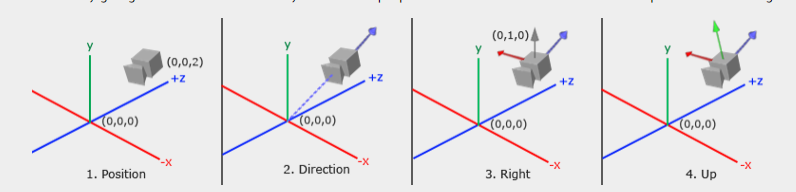### 1. Camera position

``````
glm::vec3 cameraPos = glm::vec3(0.0f, 0.0f, 3.0f);
``````

### 2. Camera direction

``````
glm::vec3 cameraTarget = glm::vec3(0.0f, 0.0f, 0.0f);
glm::vec3 cameraDirection = glm::normalize(cameraPos - cameraTarget);
``````

### 3. Right axis

``````
glm::vec3 up = glm::vec3(0.0f, 1.0f, 0.0f);
``````

### 4. Up axis

``````
``````

## Look At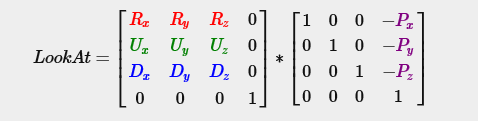``````
glm::mat4 view;
view = glm::lookAt(glm::vec3(0.0f, 0.0f, 3.0f),
glm::vec3(0.0f, 0.0f, 0.0f),
glm::vec3(0.0f, 1.0f, 0.0f));
``````

ookAt函数分别需要一个位置、目标和向上矢量。这个例子创建的视图矩阵与我们在前一章中创建的视图矩阵相同。

``````
float camX = sin(glfwGetTime()) * radius;
float camZ = cos(glfwGetTime()) * radius;
glm::mat4 view;
view = glm::lookAt(glm::vec3(camX, 0.0, camZ), glm::vec3(0.0, 0.0, 0.0), glm::vec3(0.0, 1.0, 0.0));
``````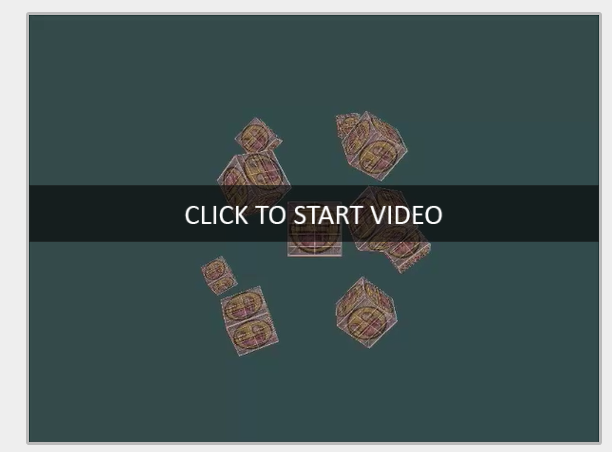# Walk around

``````
glm::vec3 cameraPos   = glm::vec3(0.0f, 0.0f,  3.0f);
glm::vec3 cameraFront = glm::vec3(0.0f, 0.0f, -1.0f);
glm::vec3 cameraUp    = glm::vec3(0.0f, 1.0f,  0.0f);
``````

LookAt函数现在变成:

``````
view = glm::lookAt(cameraPos, cameraPos + cameraFront, cameraUp);
``````

``````
void processInput(GLFWwindow *window)
{
...
const float cameraSpeed = 0.05f; // adjust accordingly
if (glfwGetKey(window, GLFW_KEY_W) == GLFW_PRESS)
cameraPos += cameraSpeed * cameraFront;
if (glfwGetKey(window, GLFW_KEY_S) == GLFW_PRESS)
cameraPos -= cameraSpeed * cameraFront;
if (glfwGetKey(window, GLFW_KEY_A) == GLFW_PRESS)
cameraPos -= glm::normalize(glm::cross(cameraFront, cameraUp)) * cameraSpeed;
if (glfwGetKey(window, GLFW_KEY_D) == GLFW_PRESS)
cameraPos += glm::normalize(glm::cross(cameraFront, cameraUp)) * cameraSpeed;
}
``````

## Movement speed

``````
float deltaTime = 0.0f;	// Time between current frame and last frame
float lastFrame = 0.0f; // Time of last frame
``````

``````
float currentFrame = glfwGetTime();
deltaTime = currentFrame - lastFrame;
lastFrame = currentFrame;
``````

``````
void processInput(GLFWwindow *window)
{
float cameraSpeed = 2.5f * deltaTime;
[...]
}
``````# Look around

## Euler angles 欧拉角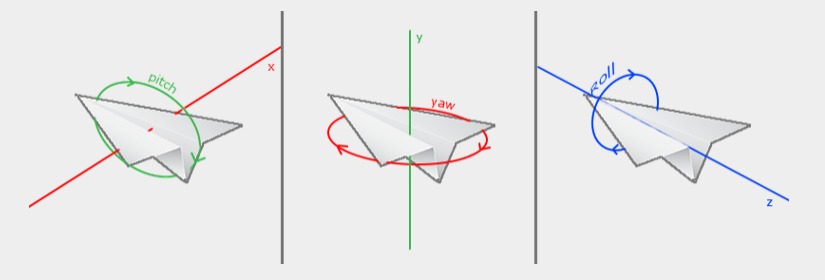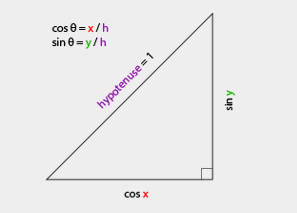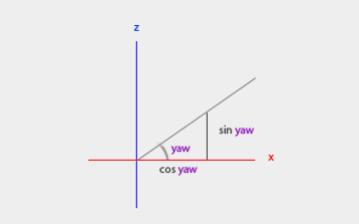``````
glm::vec3 direction;
direction.x = cos(glm::radians(yaw)); // Note that we convert the angle to radians first
``````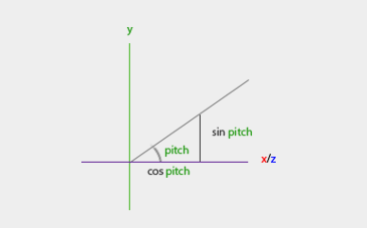``````
``````

``````
``````

``````
yaw = -90.0f;
``````

## Mouse input

``````
glfwSetInputMode(window, GLFW_CURSOR, GLFW_CURSOR_DISABLED);
``````

``````
void mouse_callback(GLFWwindow* window, double xpos, double ypos);
``````

``````
glfwSetCursorPosCallback(window, mouse_callback);
``````

1. Calculate the mouse's offset since the last frame.计算鼠标从最后一帧开始的偏移量。
2. Add the offset values to the camera's yaw and pitch values.将偏移值添加到相机的偏航和俯仰值中。
3. Add some constraints to the minimum/maximum pitch values.给最小/最大音高值添加一些约束。
4. Calculate the direction vector.计算方向向量。

``````
float lastX = 400, lastY = 300;
``````

``````
float xoffset = xpos - lastX;
float yoffset = lastY - ypos; // reversed since y-coordinates range from bottom to top
lastX = xpos;
lastY = ypos;

const float sensitivity = 0.1f;
xoffset *= sensitivity;
yoffset *= sensitivity;
``````

``````
yaw   += xoffset;
pitch += yoffset;
``````

``````
if(pitch > 89.0f)
pitch =  89.0f;
if(pitch < -89.0f)
pitch = -89.0f;
``````

``````
glm::vec3 direction;
cameraFront = glm::normalize(direction);
``````

``````
if (firstMouse) // initially set to true
{
lastX = xpos;
lastY = ypos;
firstMouse = false;
}
``````

``````
void mouse_callback(GLFWwindow* window, double xpos, double ypos)
{
if (firstMouse)
{
lastX = xpos;
lastY = ypos;
firstMouse = false;
}

float xoffset = xpos - lastX;
float yoffset = lastY - ypos;
lastX = xpos;
lastY = ypos;

float sensitivity = 0.1f;
xoffset *= sensitivity;
yoffset *= sensitivity;

yaw   += xoffset;
pitch += yoffset;

if(pitch > 89.0f)
pitch = 89.0f;
if(pitch < -89.0f)
pitch = -89.0f;

glm::vec3 direction;
cameraFront = glm::normalize(direction);
}
``````

## Zoom

``````
void scroll_callback(GLFWwindow* window, double xoffset, double yoffset)
{
Zoom -= (float)yoffset;
if (Zoom < 1.0f)
Zoom = 1.0f;
if (Zoom > 45.0f)
Zoom = 45.0f;
}
``````

``````
projection = glm::perspective(glm::radians(fov), 800.0f / 600.0f, 0.1f, 100.0f);
``````

``````
glfwSetScrollCallback(window, scroll_callback);
``````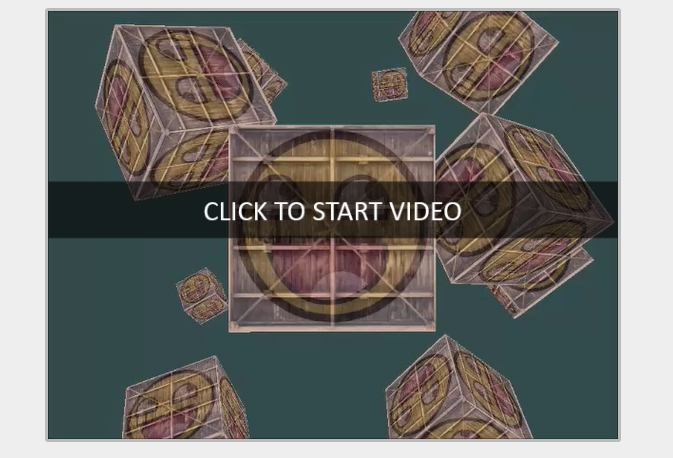# Camera class

## Exercises

• See if you can transform the camera class in such a way that it becomes a true fps camera where you cannot fly; you can only look around while staying on the `xz` plane: solution.
• Try to create your own LookAt function where you manually create a view matrix as discussed at the start of this chapter. Replace glm's LookAt function with your own implementation and see if it still acts the same: solution.